## 1.1 窗口和开窗函数简介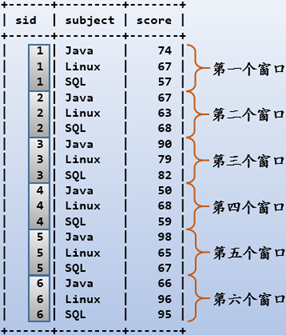## 1.2 OVER()语法和执行位置

    SELECT (11)<DISTINCT> (5)select_list (9)<OVER()>
( 1)FROM
( 3)    JOIN
( 2)    ON
( 4)WHERE
( 6)GROUP BY
( 7)WITH ROLLUP
( 8)HAVING
(10)ORDER BY
(12)LIMIT

OVER()子句的使用语法如下：

function (expression) OVER (
[ PARTITION BY expression_list ]
[ ORDER BY order_list ] )

function:
A valid window function

expression_list:
expression | column_name [, expr_list ]

order_list:
expression | column_name [ ASC | DESC ] [, ... ]

• 排名函数：ROW_NUMBER(),RANK(),DENSE_RANK(),PERCENT_RANK(),CUME_DIST(),NTILE()
• 聚合函数：COUNT(),SUM(),AVG(),BIT_OR(),BIT_AND(),BIT_XOR()
• 不支持带有DISTINCT的聚合函数，例如COUNT(DISTINCT X)

PARTITION BY子句用于划分窗口(分区)，也就是另类的GROUP BY。如果不指定PARTITION BY子句，则默认整张表是一个分区，即对所有行进行窗口函数的计算。这里的所有行不是FROM后面表的所有行，而是经过WHERE、GROUP BY、和HAVING运行之后的所有行。

## 1.3 row_number()对分区排名

row_number()函数用于给每个窗口内的行排名，且排名连续不断开的。例如，2个学生90分，一个学生89分，那么两个90分的学生将给定排名1和2，89分的学生给定排名3。至于谁是1谁是2，由物理存储顺序决定，也就是说当排名依据大小重复时，row_number()的排名将是不可预测的。

create or replace table tscore(sid int,subject char(10),score int);
insert into tscore values
(1,'Java',74),(1,'Linux',67),(1,'SQL',57),
(2,'Java',67),(2,'Linux',63),(2,'SQL',68),
(3,'Java',90),(3,'Linux',79),(3,'SQL',82),
(4,'Java',50),(4,'Linux',68),(4,'SQL',59),
(5,'Java',98),(5,'Linux',65),(5,'SQL',67),
(6,'Java',66),(6,'Linux',96),(6,'SQL',95);
select * from tscore;
+------+---------+-------+
| sid  | subject | score |
+------+---------+-------+
|    1 | Java    |    74 |
|    1 | Linux   |    67 |
|    1 | SQL     |    57 |
|    2 | Java    |    67 |
|    2 | Linux   |    63 |
|    2 | SQL     |    68 |
|    3 | Java    |    90 |
|    3 | Linux   |    79 |
|    3 | SQL     |    82 |
|    4 | Java    |    50 |
|    4 | Linux   |    68 |
|    4 | SQL     |    59 |
|    5 | Java    |    98 |
|    5 | Linux   |    65 |
|    5 | SQL     |    67 |
|    6 | Java    |    66 |
|    6 | Linux   |    96 |
|    6 | SQL     |    95 |
+------+---------+-------+

select
row_number() over(partition by sid order by score desc) as rnum,
sid,
subject,
score
from tscore;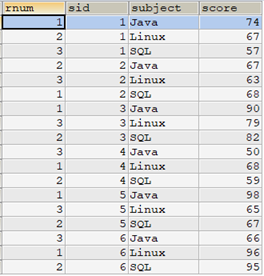select
row_number() over(partition by sid order by score desc) as rnum,
tscore.*
from tscore
order by sid,rnum;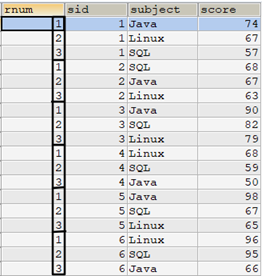select
/* 使用row_number() */
row_number() over(partition by t1.sid order by t1.score desc) as rnum1,
/* 使用子查询 */
(select count(*)+1 from tscore t2 where t2.sid=t1.sid and t2.score>t1.score) as rnum2,
t1.*
from tscore t1
order by sid,rnum1;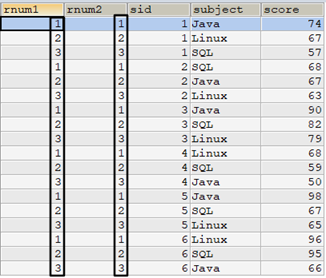/* 给定score重复的值 */
create table tscore_tmp like tscore;
insert into tscore_tmp select * from tscore;
update tscore_tmp set score=67 where sid=1 and subject='Java';

select
/* 使用row_number() */
row_number() over(partition by t1.sid order by t1.score desc) as rnum1,
/* 使用子查询 */
(select count(*)+1 from tscore_tmp t2 where t2.sid=t1.sid and t2.score>t1.score) as rnum2,
t1.*
from tscore_tmp t1
order by sid,rnum1;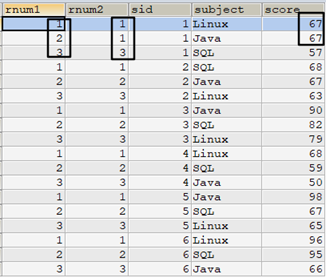select
/* 使用row_number() */
row_number() over(partition by t1.sid order by t1.score desc) as rnum1,
/* 使用子查询 */
(select count(*)+1 from tscore_tmp t2
where t2.sid=t1.sid
and (t2.score>t1.score
or  (t2.score=t1.score and t2.subject>t1.subject))) as rnum2,
t1.*
from tscore_tmp t1
order by sid,rnum1;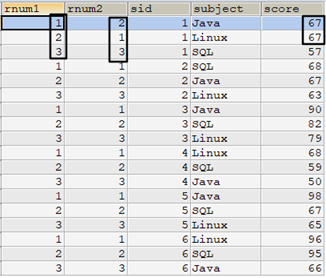## 1.4 rank()和dense_rank()

select t.*,
/* 使用ROW_NUMBER()的方式排名 */
row_number() over(partition by sid order by score desc) as rnum,
/* 使用RANK()的方式排名 */
rank() over(partition by sid order by score desc) as rank,
/* 使用DENSE_RANK()的方式排名 */
dense_rank() over(partition by sid order by score desc) as dense_rank
from tscore_tmp t
order by t.sid,rnum;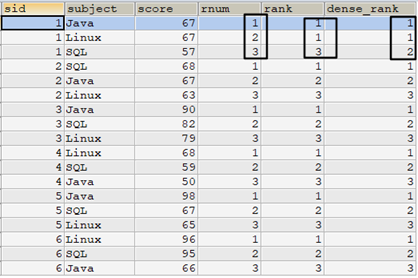select t1.*,
/* 使用ROW_NUMBER()的方式排名 */
(select count(*) + 1 from tscore_tmp AS t2
where t2.sid = t1.sid
and  (t2.score > t1.score
or   (t2.score = t1.score and t2.subject > t1.subject))) as rnum,
/* 使用RANK()方式排名 */
(select count(*) + 1 from tscore_tmp t2
where t2.sid = t1.sid
and   t2.score > t1.score) as rank,
/* 使用DENSE_RANK()方式排名 */
(select count(distinct score) + 1 from tscore_tmp t2
where t2.sid = t1.sid
and   t2.score > t1.score) as dense_rank
from tscore_tmp t1
order by sid,rnum;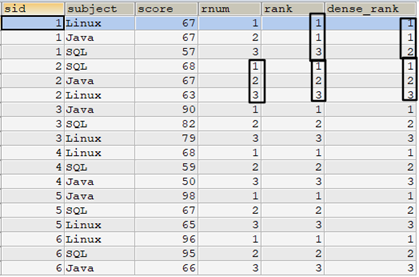## 1.5 percent_rank()和cume_dist()

percent_rank()函数用于计算分组中某行的相对排名。

cume_dist()函数用于计算分组中某排名的相对比重。

percent_rank()的计算方式：(窗口中rank排名-1)/(窗口行数-1)
cume_dist()的计算方式：(窗口中小于或等于当前行的行数)/窗口行数，即(<=当前rank值的行数)/窗口行数

select
t1.*,
row_number() over(partition by sid order by score desc) as rnum,
rank() over(partition by sid order by score desc) as rank,
percent_rank() over(partition by sid order by score desc) as pct_rank,
cume_dist() over(partition by sid order by score desc) as cume_dist
from tscore_tmp t1
order by sid,rnum;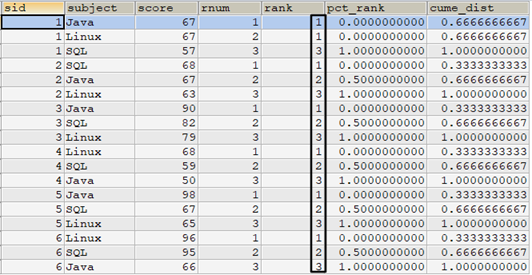- pct_rank列是根据每个窗口中，rank列的值计算的。例如sid=1,score=67的两行记录，它们的rank值都是1，窗口中的行数位3，所以计算方式为(1-1)/(3-1)=0。

- cume_dist列计算的是每个窗口中某个rank值所占排名比重。例如sid=1,score=67的两行记录，这两行的rank值都为1，窗口中3行，所以计算方式为2/3=0.67。

select
t1.*,
row_number() over(order by score desc) as rnum,
rank() over(order by score desc) as rank,
percent_rank() over(order by score desc) as pct_rank,
cume_dist() over(order by score desc) as cume_dist
from tscore_tmp t1
order by rnum;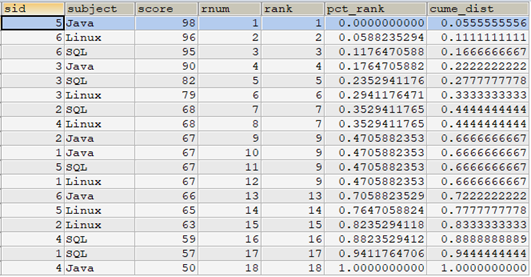- pct_rank=(9-1)/(18-1)=8/17=0.47

- cume_dist=12/18=0.67

## 1.6 ntile()数据分组

NTILE()的功能是进行"均分"分组，括号内接受一个代表要分组组数量的参数，然后以组为单位进行编号，对于组内每一行数据，NTILE都返回此行所在组的组编号。简单的说就是NTILE函数将每一行数据关联到组，并为每一行分配一个所属组的编号。

SELECT
tscore.*,
row_number() over (ORDER BY score) AS rnum,
ntile(4) over (ORDER BY score) AS ntile
FROM    tscore
ORDER BY rnum;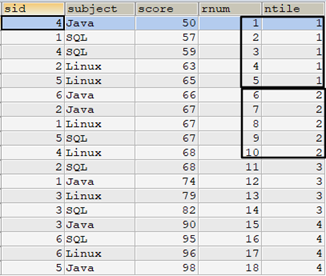select
t.*,
row_number() over(partition by subject order by score desc) as rnum,
case ntile(2) over(partition by subject order by score desc)
when 1 then 'higher score'
when 2 then 'lower score'
end as ntile
from tscore t
order by subject,rnum;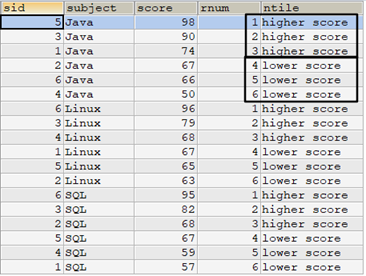LAG (expr[, offset]) OVER (
[ PARTITION BY partition_expression ]
< ORDER BY order_list >
)

[ PARTITION BY partition_expression ]
[ ORDER BY order_list ]
)

select
t.subject,t.score,
row_number() over(partition by subject order by score desc) as rnum,
lag(sid,1) over(partition by subject order by score desc) as lag,
t.sid,
from tscore t
order by subject,rnum;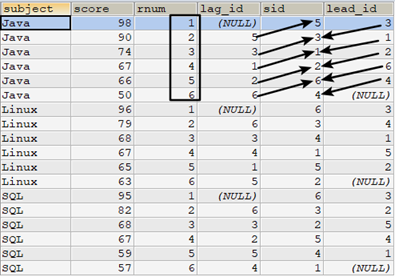## 1.8 窗口聚合函数

OVER([PARTITION BY expression])

OVER()括号内的内容省略时表示对所有筛选后的行数据进行聚合。

select
t.*,
avg(score) over() as sum_avg,
avg(score) over(partition by sid) as sid_avg
from tscore t;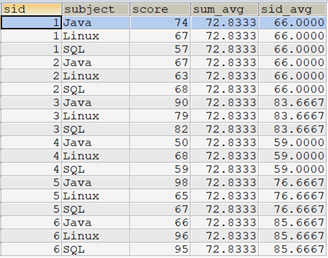select t1.*,t2.sum_avg
from tscore t1
cross join
(select avg(score) as sum_avg from tscore) as t2;

select t1.*,t3.sid_avg
from tscore t1
left join (select sid,avg(score) as sid_avg from tscore
group by sid) as t3
on t3.sid=t1.sid;

select t1.*,t2.sum_avg,t3.sid_avg
from tscore t1
cross join (select avg(score) as sum_avg from tscore) t2
left join (select sid,avg(score) as sid_avg from tscore
group by sid) as t3
on t1.sid=t3.sid;

select t1.*,
avg(score) over() as sum_avg,
score - avg(score) over() as diff_avg
from tscore t1;

/* 使用联接的方式 */
select t1.*,sum_avg,score - t2.sum_avg as diff_avg
from tscore t1
cross join (select avg(score) as sum_avg from tscore) as t2;

SELECT t1.*,
AVG(score) OVER(PARTITION BY sid)
FROM tscore t1

/* 下面的查询和上面的查询性能一样 */
SELECT t1.*,
AVG(score) OVER(PARTITION BY sid) AS Avgscore,
SUM(score) OVER(PARTITION BY sid) AS Sumscore,
MAX(score) OVER(PARTITION BY sid) AS Maxscore,
MIN(score) OVER(PARTITION BY sid) AS Minscore
FROM tscore t1;

select t1.*,Avgscore,Sumscore,Maxscore,Minscore
from tscore t1
left join (select sid,
AVG(score) Avgscore,
SUM(score) Sumscore,
MAX(score) Maxscore,
MIN(score) Minscore
from tscore
group by sid) as t2
on t1.sid=t2.sid;

## 1.9 开窗函数的性能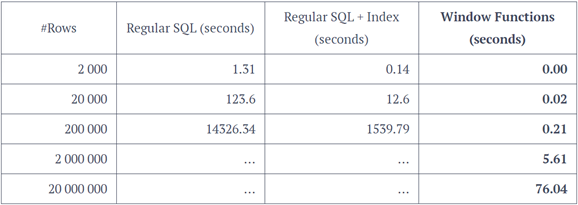posted @ 2018-04-25 15:50  骏马金龙  阅读(...)  评论(...编辑  收藏